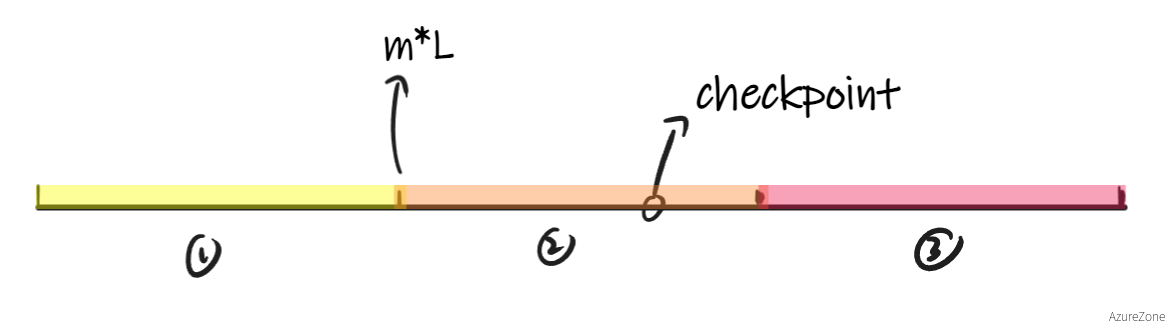### Index和Seqno的转换

#### 代码思路

##### checkpoint > L

$\left ( index+isn \right )\mod{ L } = seqno$

$index = seqno + k * L - isn$

$m = checkpoint / L$

m作为一个附近值，通过画图可以知道，在一般情况下，答案一定在checkpoint附近的三个区间内###### seqno - isn > 0

seqno - isn为正数的时候，index可能的一个取值会落在第②个区间上，有

$index'' = m * L + seqno - isn$

$index' = index'' - L \\\\ index''' = index'' + L$

index'index''index'''的中间值进行判断，很容易得到以下规律

$\begin{array}{l} 令a = m*L+seqno-isn \\\\ L = UINT32\_MAX+1 \\\\ \left \{\begin{matrix} checkpoint < a - L/2 & \text{index=a-L}\\\\ a-L/2\leq checkpoint< a+L/2 & \text{index=a}\\\\ a+L/2\leq checkpoint & \text{index=a+L} \end{matrix}\right. \end{array}$

###### seqno - isn < 0

$index' = m * L + seqno - isn \\\\ index'' = index' + L \\\\ index''' = index' + 2 * L$

$\begin{array}{l} 令a = m*L+seqno-isn \\\\ L = UINT32\_MAX+1 \\\\ \left \{\begin{matrix} checkpoint < a + L/2 & \text{index=a}\\\\ a+L/2\leq checkpoint< a+（3*L）/2 & \text{index=a + L}\\\\ a+（3*L）/2\leq checkpoint & \text{index=a+2*L} \end{matrix}\right. \end{array}$

$\begin{array}{l} 令a = m*L+seqno-isn \\\\ L = UINT32\_MAX+1 \\\\ \left \{\begin{matrix} checkpoint < a - L/2 & \text{index=a-L}\\\\ a-L/2\leq checkpoint< a+L/2 & \text{index=a}\\\\ a+L/2\leq checkpoint< a+（3*L）/2 & \text{index=a + L}\\\\ a+（3*L）/2\leq checkpoint & \text{index=a+2*L} \end{matrix}\right. \end{array}$

##### checkpoint < L

checkpoint < L的时候，通解中对于a - L的一部分（即checkpoint < a + L）就不适用了，不过分析起来也很简单，由于有

$\left ( index+isn \right )\mod{ L } = seqno$

### TCP 段接收处理

• _is_syn: 判断链接是否建立
• _isn: 存入第一次建立连接时接受的seqno来初始化
• _is_fin: 用于判断结束输入的报文是否传入

• ackno: 本质上就是返回已经整合好的数据量，也就是bytes_streambytes_written()，同时建立连接后一定存在syn所以可以直接加一，之后只需要判断fin是否到达并且整合完毕，然后再次加一即可。
• checkpoint: 和ackno差别不大，只需要直接返回已经写入完成的字符个数即可

• 存在同时携带SYNFIN报文，按照正常的TCP握手感觉这是不合理的
• 在接受SYN的同时会接受一部分的Data进行处理，按正常的TCP也是不会这么做的

https://halc.top/p/4e68707

HalcyonAzure

2022年11月22日